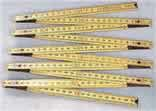Definition of

# Milli-A prefix meaning one-thousandth (1/1,000 or 10-3)

Example: a millimeter is 1/1,000th of a meter (in other words 1,000 millimeters make 1 meter)

Symbol is m (as a prefix, otherwise "m" means meter)

Examples:
12 ms = 12 milliseconds = 12 thousandths of a second (0.012 s)
95 mm = 12 millimeters = 95 thousandths of a meter (0.095 m)
See: Centi-